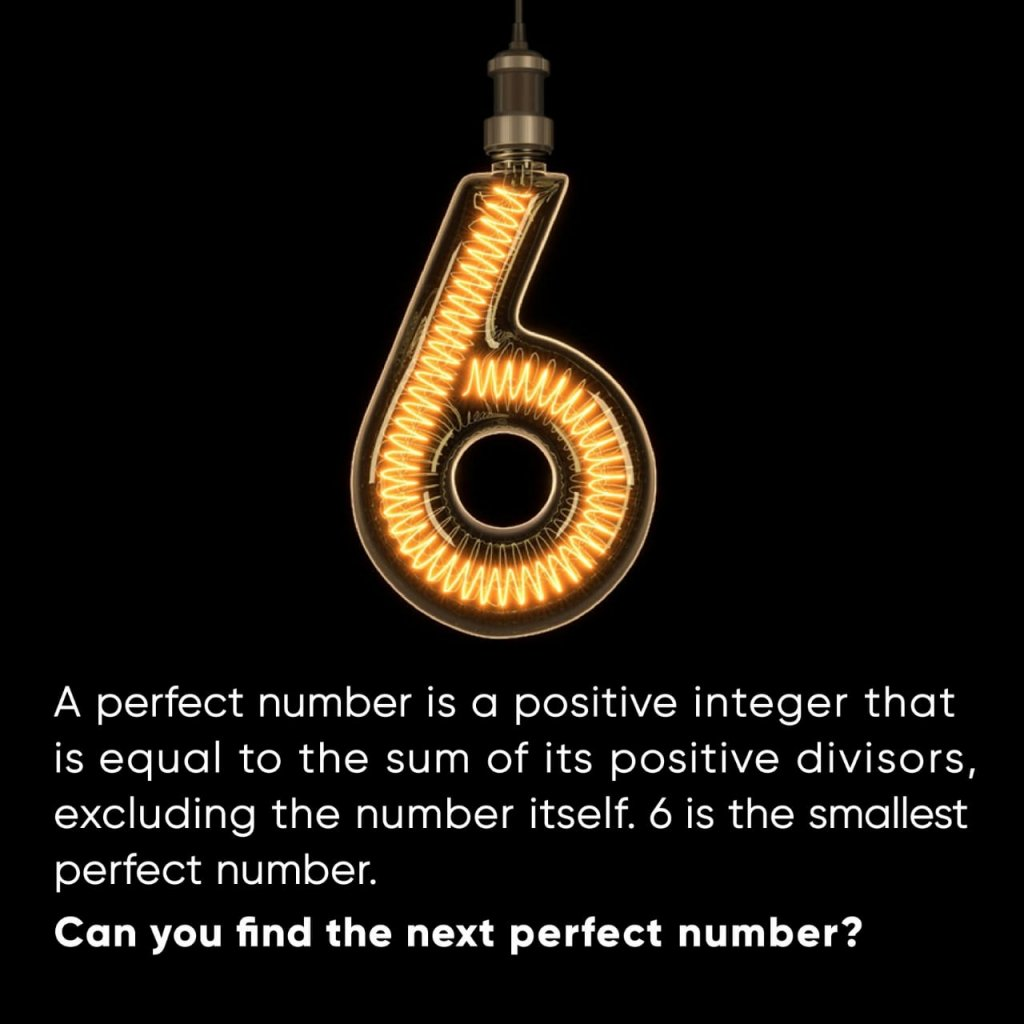Math Concepts

# Mystical Math – The Magic of Perfect Numbers1 What is a perfect number? 2 What is the smallest perfect number? 3 Theory of Perfect Numbers

## What is a perfect number?

In number theory, a perfect number is a positive integer that is equal to the sum of its positive divisors, excluding the number itself. The discovery of such numbers is lost in prehistory. Pythagoreans (founded c. 525 BCE) studied perfect numbers for their “mystical” properties.

## What is the smallest perfect number?

The smallest perfect number is 6 – it has divisors 1, 2 and 3 (excluding 6) and:

 6 = 1 + 2 + 3

## Theory of Perfect Numbers

However, you need not worry beyond a point because about 2000 years ago, Euclid came up with a handy algorithm for finding the perfect numbers. Mathematically, his proposition can be stated as: Now, if you are worried about finding the next perfect number using only the definition, then you are not alone! It is indeed a daunting task.

If, for some n>1,  \begin{align}{2^{\{ n \} }-1}\end{align} is a prime number, then:

(2-1)*(2n-1) is a perfect number.

Now let’s start testing this algorithm starting with the value of n as 2.

For n=2,

2n-1=22-1=3 ( prime)

2n-1=22-1=21=2

Therefore,

\begin{align}\left( {{2^{n - 1}}} \right) \times \left( {{2^n} - 1} \right) = 2 \times 3 = 6\end{align}

We have already shown that 6 is a perfect number. So the algorithm works for n=2.

For n=3,

2n-1=23-1=7 (prime)

2n-1=23-1=22=4

Therefore,

\begin{align}\left( {{2^{n - 1}}} \right) \times \left( {{2^n} - 1} \right) = 4 \times 7{\rm{ }} = 28\end{align}

Hence, according to the algorithm, 28 should be the next perfect number.

But we need to verify this using the definition of perfect numbers:

The divisors of 28 are 1,2,4,7 and 14.(Excluding the number itself)

And 28 can be written as:

 28 = 1 + 2 + 4 + 7 + 14

Voila! 28 is indeed a perfect number.

So the algorithm works for n=3.

So here we are! We now have our 2nd perfect number which is 28. We used the algorithm to arrive at this number and verified that it is perfect by using the definition of perfect numbers.

Now, can you find the next two perfect numbers? (Pro Tip: Try those values of n which are prime; for example, when n is equal to 5, 7 11, or 13….)

## Conclusion

Hope you enjoyed this post! Cuemath, a student-friendly mathematics platform, conducts regular Online Live Classes for academics and skill-development and their Mental Math App, on both iOS and Android, is a one-stop solution for kids to develop multiple skills.